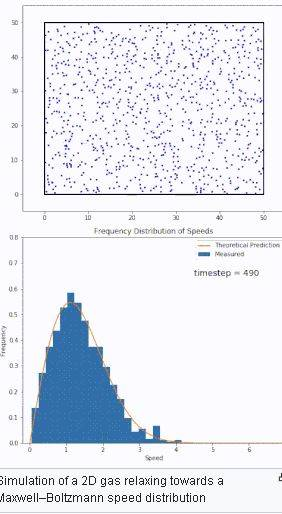# Is the Boltzmann energy distribution an instance of energy diffusion?

SteveMaryland
I (mechanical engineer) have researched this question but can't get to an answer.

The equilibrium condition for confined particle diffusion of a solute in a solvent is reached when the solute spatial density is uniform (= zero density gradient), and entropy is max.

But per Boltzmann, when confined particles reach equilibrium, a non-zero energy gradient) persists indefinitely, even as max entropy is reached.

My assumption here is that both are cases of diffusion - one of species, one of thermal energy.

If both processes are cases of diffusion, why, at max entropy, does solute diffusion reach a zero density gradient, but energy diffusion reaches a non-zero energy gradient?

Homework Helper
Hi,

But per Boltzmann, when confined particles reach equilibrium, a non-zero energy gradient) persists indefinitely, even as max entropy is reached.
Could you clarify this ? What gradient are you referring to ?

##\ ##

SteveMarylandBoltzmann predicts that a non-zero range (= distribution, = gradient) of particle energies will persist "forever" in the steady-state. Since this is an energy-diffusion process, why do the particle energies not "diffuse" to a single uniform value for all particles the same way a solute/solvent particle density gradient diffuses to zero in the steady-state?

Homework Helper
non-zero range (= distribution, = gradient)
That is not correct. A non-zero gradient means that there is a change when you look at another position.

The distribution as found comes out as a result of averaging and is not position dependent: the outcome is the same in all places. Ergo a zero gradient.

##\ ##

Gold Member
2022 Award
But isn't this a pretty simple outcome of the diffusion equation,
$$\partial_t \rho(t,\vec{x})=D \Delta \rho(t,\vec{x}).$$
Solving this for a finite volume with the appropriate boundary conditions, this gives ##\rho=\text{const}## for ##\partial_t \rho=0##.

•dextercioby
SteveMaryland
That is not correct. A non-zero gradient means that there is a change when you look at another position.

The distribution as found comes out as a result of averaging and is not position dependent: the outcome is the same in all places. Ergo a zero gradient.

##\ ##
What then is the "heat death" of the universe? My understanding of the "heat death" is that condition reached where all of the thermal energy in the universe has (spontaneously) diffused to the point where there are no more (= zero) thermal gradients anywhere - all thermal energy has diffused to the limit of uniform distribution, just like ink will (spontaneously) diffuse in a solution to the limit of completely uniform distribution.

To what extent does the universe resemble the Maxwell-Boltzmann box of particles?

If there IS a resemblance, then that means that thermal energy will never "completely" diffuse (like ink in water) but rather will "stop" at the M-B distribution - which in turn means there will always be vestigial thermal gradients in the universe and "heat death" will never be reached.

Homework Helper
You have lost me completely. And it seems to me you still think that there is a gradient where there is none.

##\ ##

SteveMaryland
Alright, I will restate the question without using the word gradient - it's the same question:

What then is the "heat death" of the universe? My understanding of the "heat death" is that condition reached where all of the thermal energy in the universe has (spontaneously) diffused to the limit of uniform distribution, just like ink will (spontaneously) diffuse in a solution to the limit of completely uniform distribution.

To what extent does the universe resemble the Maxwell-Boltzmann box of particles? If there IS a resemblance, then that means that thermal energy will never "completely" diffuse (like ink in water) but rather will "stop" at the M-B distribution - which in turn means there will always be vestigial thermal gradients differences in the universe and "heat death" will never be reached.